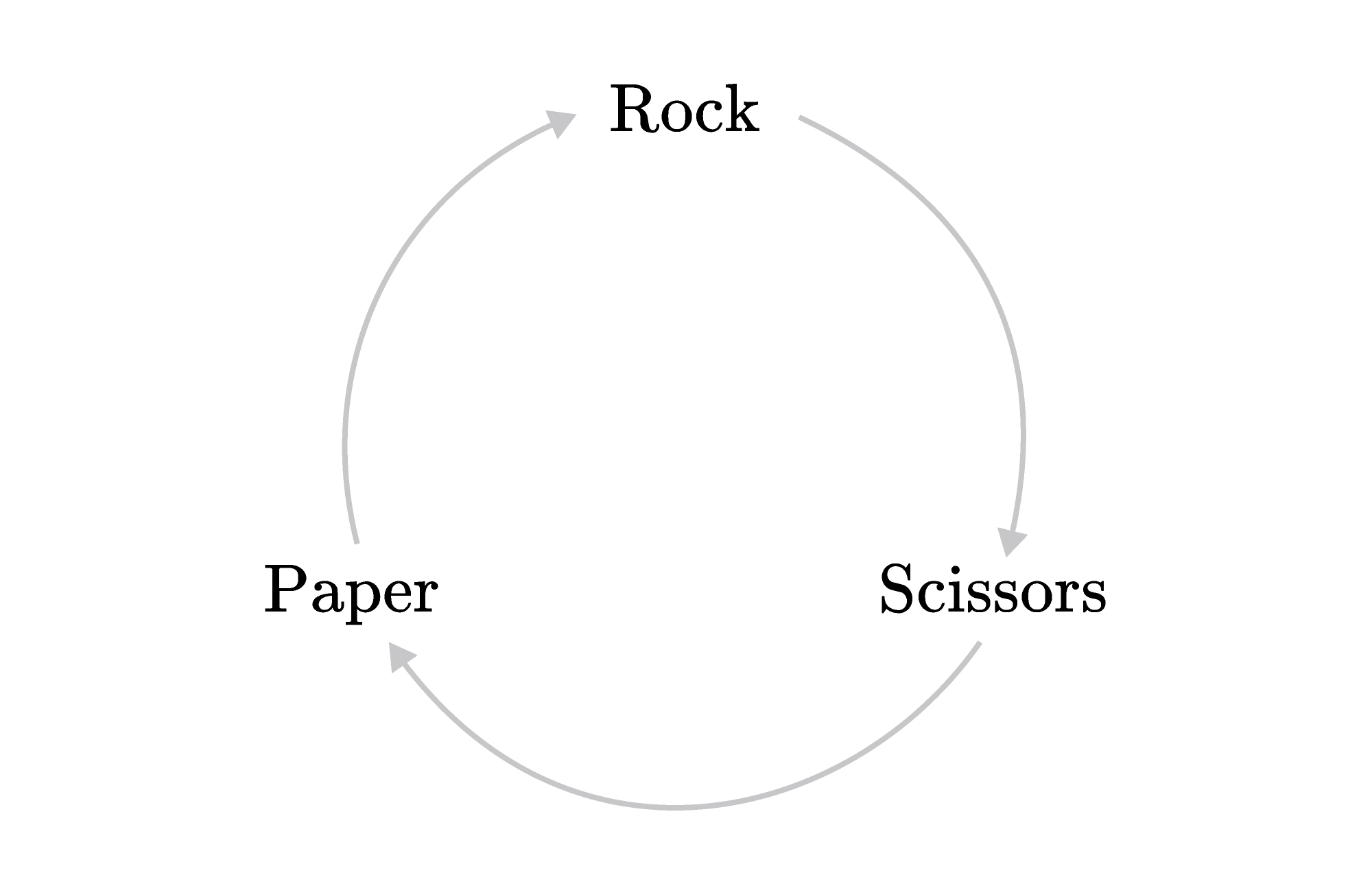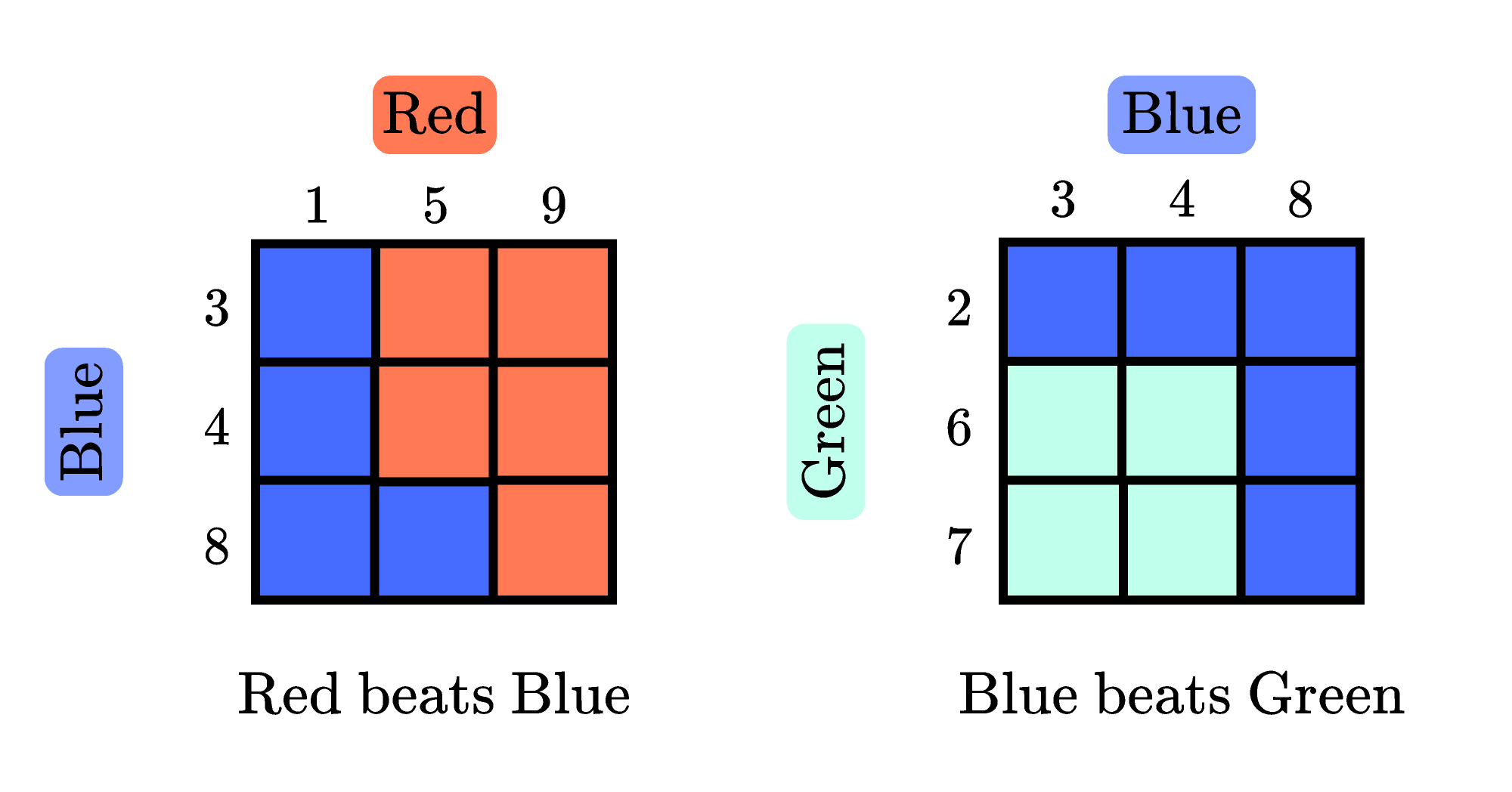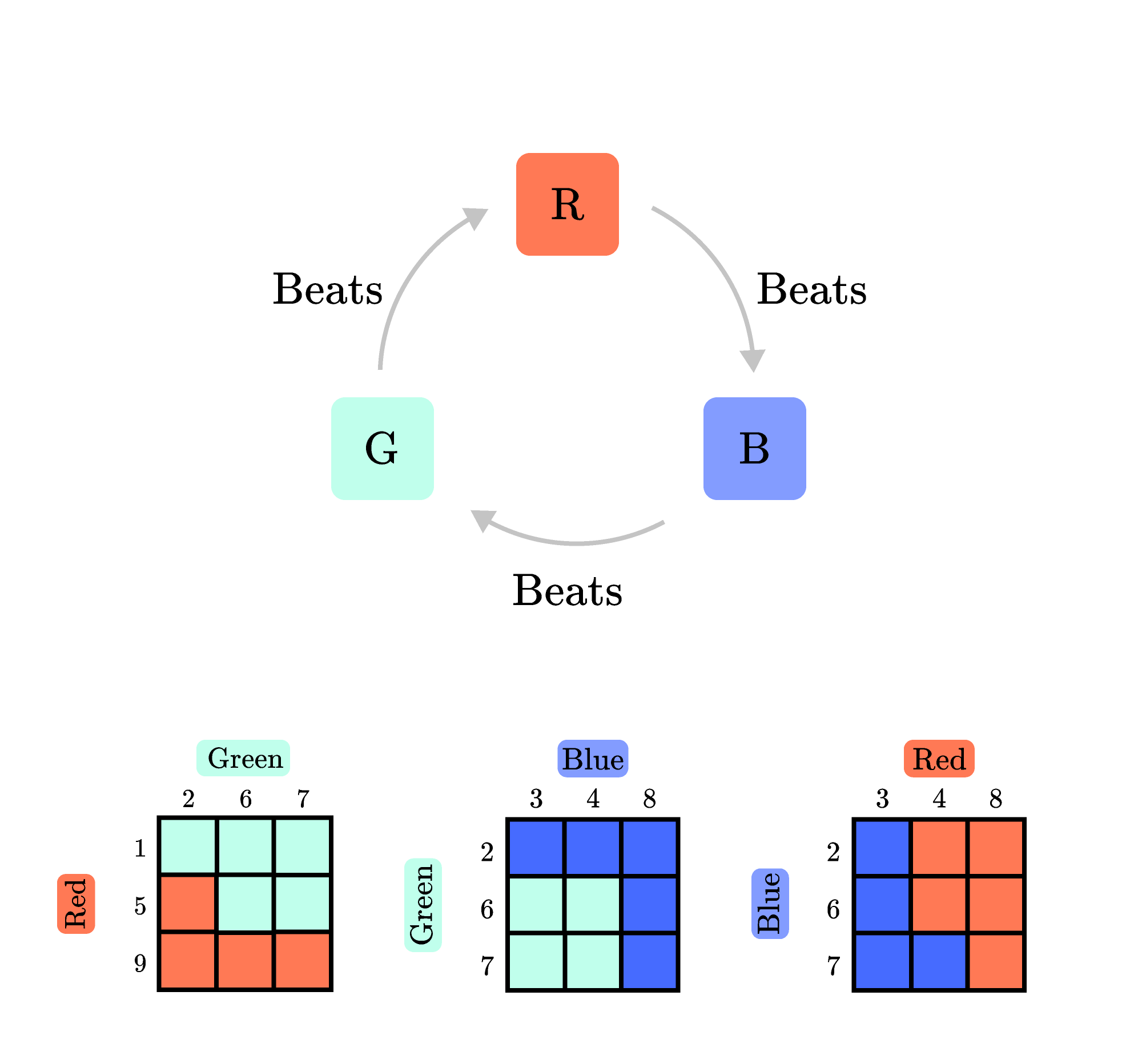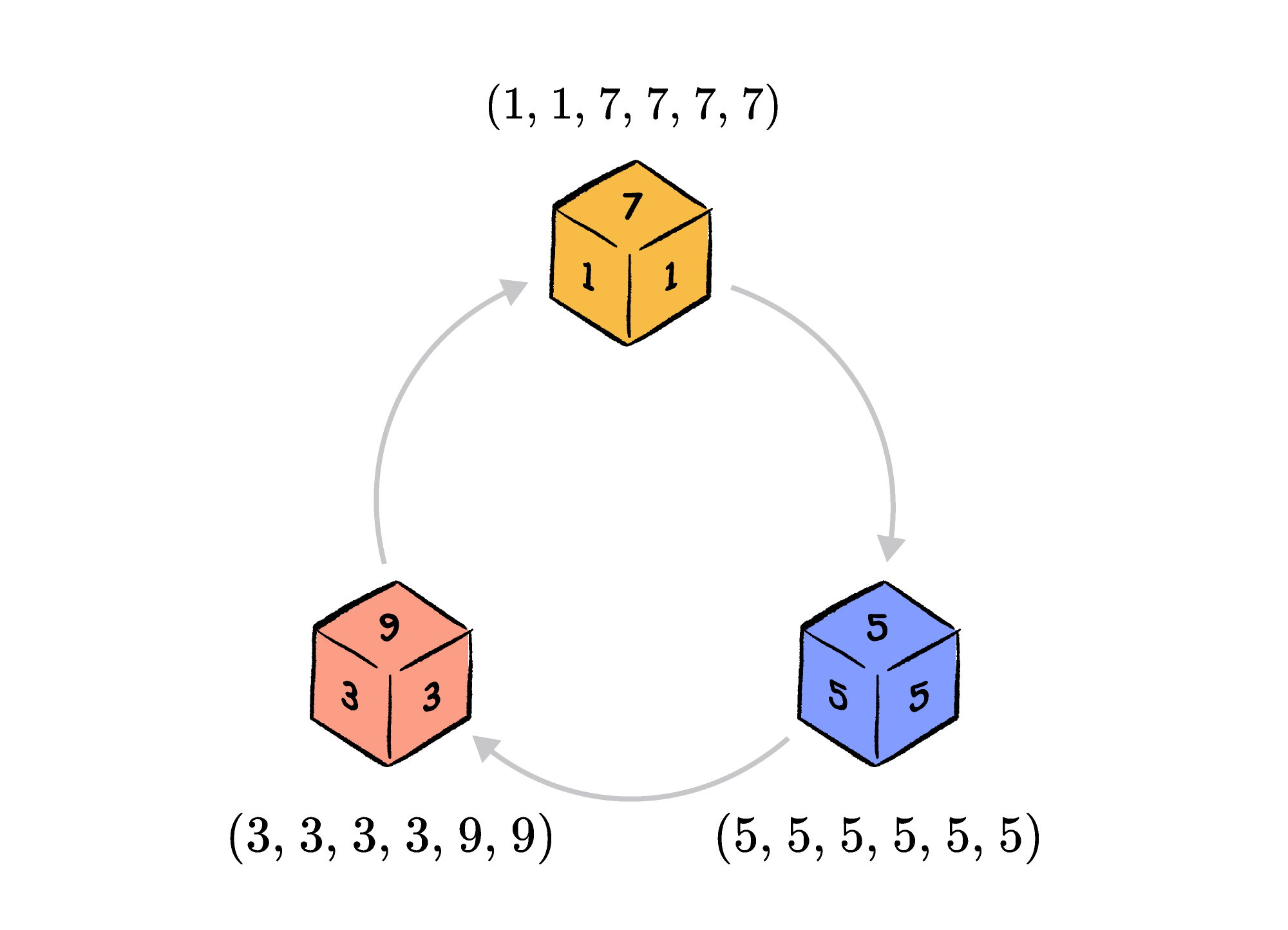Back

## Non-transitive Dice

If Alice is taller than Bob and Bob is taller than Calvin, what can we say about Alice and Calvin? We don’t need to see Alice standing next to Calvin to know which one is taller.

However, this principle of ordering isn’t universal. Consider the game Rock, Paper, Scissors. Rock beats Scissors (by smashing them), and Scissors beats Paper (by cutting it). If we applied the same logic to this game that we applied to the heights above, we’d say that Rock beats Paper. Instead, by the rules of the game, Paper beats Rock (by covering it).

Heights are numerical, while the moves of Rock, Paper, Scissors are not. Can our typical assumption about ordering be broken when numbers are involved? To see an example, keep reading; or, jump ahead straight to today's challenge.

Heights are transitive, which is why we can conclude that Alice is taller than Calvin. If the moves in Rock, Paper, Scissors were transitive as well, we would have a chain of moves ordered by strength: $\text{Rock} \; \succ \; \text{Paper} \; \succ \; \text{Scissors}.$ But Rock, Paper, Scissors is nontransitive. Rather than a chain of moves, we have a cycle of moves, with no overall dominant strategy:This concept can arise with dice as well. Consider a game where we roll two dice and whichever one lands on a higher number wins that round. Suppose we have three different three-sided dice:

• Red, with sides numbered $1, 5, 9$
• Blue, with sides numbered $3, 4, 8$
• Green, with sides numbered $2, 6, 7.$

By organizing the possible outcomes in a table, we can visualize how often one die might roll a higher number than another:Comparing the Red and Blue dice: Red wins whenever a $9$ is rolled, it loses whenever a $1$ is rolled, and it wins $\frac 2 3$ of the time when a $5$ is rolled. Every square in the table is equally likely, so the probability that Red rolls a higher number than Blue is $\frac 5 9.$ In any given round, it’s more likely that Red wins, so we say that Red "beats" Blue. Using the same reasoning, we can see that Blue beats Green.

However, even though Red beats Blue and Blue beats Green, it's actually the case that Green beats Red! This dice game and this set of dice are non-transitive, just like Rock, Paper, Scissors.Is it possible to add a fourth die to this set and have it remain non-transitive? You can try in the challenge below with six-sided dice.

# Today's Challenge

Consider a game played with the three dice shown below. Two dice are rolled, and whichever lands on a higher number wins the round. Many rounds of this game will be played, so we want to pick the die most likely to win in the long term.

Which one should we pick? Sadly, there is no overall winning die in this set. For example, over many rounds, Yellow versus Blue results in Yellow winning, but Yellow loses when played against Red.Now, suppose we add a fourth die to the set of three above. Which of the following dice could be added so every die still loses to at least one other and also beats at least one other?

Note: An equal probability of winning and losing does not count as winning or losing for this problem.×